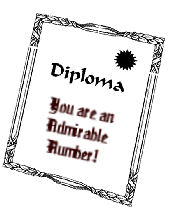Admirable Numbers[NOTE: The information in this page is based on an article by J. M. Sachs. Admirable Numbers and Compatible Pairs. The ARITHMETIC TEACHER, October 1960. pp. 293-5]

In two other articles we discussed the well known ideas of perfect, deficient and abundant numbers and amicable numbers. These categories are famous and often discussed in books on number theory. But one day long ago I found an article written by a math teacher in which he described a new category of number, using the same basic idea of the sum of the factors of a number. He called it Admirable Numbers.

Recall for a moment what makes a perfect number: the sum of the proper factors of a number must equal the number itself. And as noted earlier, like the rarity of perfect people in the world, there are not many perfect numbers either. But, you know? There are surely some people who are, at least, admirable, right? Well, I think, Mr. Sachs must have thought so, too.

He defines an admirable number in this fashion: if the sum of all the proper factors, except one of them, are added, and that final one is subtracted, the original number will result. An example will serve to clarify this.

12 is an admirable number because:

1. its proper factors are 1, 2, 3, 4, and 6; and

2. 12 = 1 + 3 + 4 + 6 - 2.

The point: we couldn't use all factors as addends as is done for perfect numbers. The "flaw" is that subtraction had to be used to achieve the "sum" of 12. But one little flaw isn't that bad in one's character; we all have at least one aspect of our lives we could improve on. Hence, why not call "12" sort of admirable after all?

It should be obvious that as there are more admirable people in the world, there should be more admirable numbers as well. But even at that, they are not omnipresent either. To be admirable, a number must first be abundant, else there would be insufficient quantity to subtract. For example, 18 is an abundant number. Its proper factors are 1, 2, 3, 6, and 9; they have a sum of 21. Yet it is not admirable. Observe:

• 1 + 2 + 3 + 6 - 9 = 6

• 1 + 2 + 3 + 9 - 6 = 9

• 1 + 2 + 6 + 9 - 3 = 12

• 1 + 3 + 6 + 9 - 2 = 17

• 2 + 3 + 6 + 9 - 1 = 19

So while a number must be abundant in order to be admirable, that in no way guarantees that it is admirable. (Can you begin to see the connection here with the world of humanity as well?)

The search is now before you: (1) find some abundant numbers; then (2) in addition, determine which of them are admirable.

Mr. Sachs went one step further. Using the analogy of amicable numbers, he defined something called compatible numbers. In this case, an example will more easily serve us instead of a complicated definition. 30 and 40 are compatible because

30: 2 + 3 + 5 + 6 + 10 + 15 - 1 = 40

and

40: 1 + 2 + 4 + 5 + 8 + 20 - 10 = 30.

You see? The proper factors of 30 make an admirable expression equaling 40, and the proper factors of 40 make an admirable expression that equals 30. Nice, neat, and just what it should be.

Now, can you find more admirable numbers and compatible pairs?

Happy hunting.